3.G.A.2 Test 7D Partition shapes into parts with equal areas.
 Name:    3.G.A.2 Test 7D Partition shapes into parts with equal areas.

Multiple Choice
Identify the choice that best completes the statement or answers the question.

1.

Which fraction below describes how this rectangle is divided?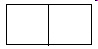a. 2/3 c. 2/2 b. 1/2 d. 1/4

2.

Which fraction below describes how this rectangle is divided?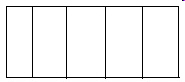a. 1/5 c. 1 b. 5/5 d. 5/1

3.

Which fraction below describes how this rectangle is divided?a. 1/8 c. 4/1 b. 4 d. 1/4

4.

Which fraction below describes how this rectangle is divided?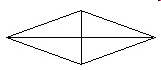a. 4 c. 1/4 b. 4/1 d. 1/8

5.

Which fraction below describes how this rectangle is divided?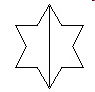a. 1/4 c. 2/2 b. 2/1 d. 1/2

6.

Which fraction below describes how this rectangle is divided?a. 1/4 c. 1/2 b. 1/8 d. 8/1

7.

Which fraction below describes how this rectangle is divided?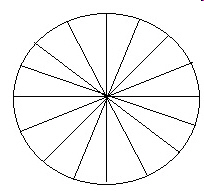a. 8/16 c. 1/16 b. 16/1 d. 1/8